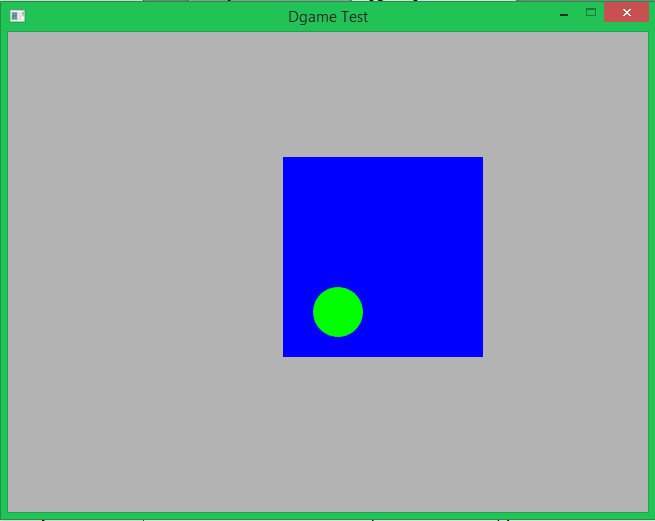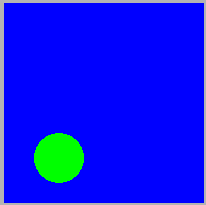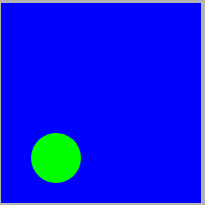# OpenGL Settings : Anti Aliasing

As you may have noticed, if you draw Shapes (especially circles) the edges are not smooth. That's because anti aliasing is disabled as default option because anti aliasing is a very costly option which draws nice and smooth lines but decreases the framerate. But nevertheless, if you want to use it, here is how to do it:

Show Raw
```import std.stdio;

import Dgame.Window;
import Dgame.Graphic;
import Dgame.Math;
import Dgame.System;

void main() {
Window wnd = Window(640, 480, "Dgame Test", Window.Style.Default, GLSettings(0, 0, 8));
wnd.setVerticalSync(Window.VerticalSync.Enable);
wnd.setClearColor(Color4b.Gray);

Shape qs = new Shape(Geometry.Quad,
[
Vertex( 75,  75),
Vertex(275,  75),
Vertex(275, 275),
Vertex( 75, 275)
]
);

qs.fill = Shape.Fill.Full;
qs.setColor(Color4b.Blue);
qs.setPosition(200, 50);

Shape circle = new Shape(25, Vector2f(180, 380));
circle.setColor(Color4b.Green);
circle.setPosition(150, -100);

bool running = true;

Event event;
while (running) {
wnd.clear();

while (wnd.poll(&event)) {
switch (event.type) {
case Event.Type.Quit:
writeln("Quit Event");

running = false;
break;

case Event.Type.KeyDown:
if (event.keyboard.key == Keyboard.Key.T) {
if (qs.geometry == Geometry.Quad)
qs.geometry = Geometry.Triangle;
else
} else if (event.keyboard.key == Keyboard.Key.Space)
circle.move(4, -4);
break;

default: break;
}
}

wnd.draw(qs);
wnd.draw(circle);

wnd.display();
}
}```

That should result in something like this:You may note the the GLSetting struct here

```Window(640, 480, "Dgame Test", Window.Style.Default, GLSettings(0, 0, 8));
```

which is used with the parameters 0, 0 and 8.
The first parameters is the major version of OpenGL you want to use. A value of 0 means "get the highest supported on my machine". The second parameter is the minor version of OpenGL and again a value of 0 means "get the highest possible". If you want to get a lower version you can specify that with these both.

The third option is the anti-aliasing level. Higher levels result in nicer smooth lines, but you should also be aware that this is a very expensive operation that reduces the frame rate drastically.

## ComparisonAnti-aliasing level of 0Anti-aliasing level of 8